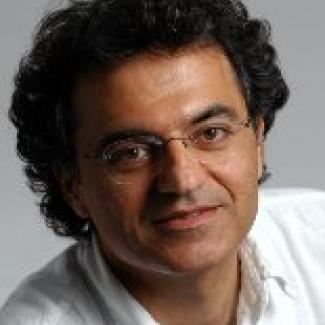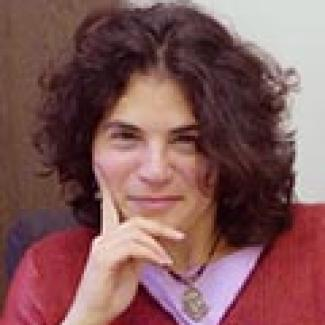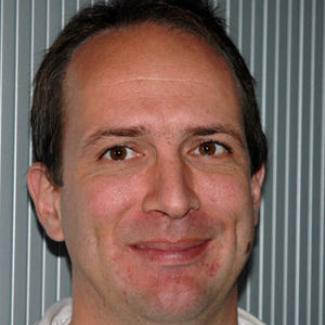Quantum Hamiltonian complexity is an exciting area combining deep questions and techniques from both quantum complexity theory and condensed matter physics. The connection between these fields arises from the close relationship between their defining questions: the complexity of constraint satisfaction problems in complexity theory and the properties of ground states of local Hamiltonians in condensed matter theory. At one end of the spectrum, quantum Hamiltonian complexity expands the scope of computational complexity theory in new directions by asking three fundamental questions:

• Can ground states of “natural” quantum systems be described succinctly?
• Does the exponential complexity of general quantum systems persist at high temperature?
• Is the scientific method sufficiently powerful to understand general quantum systems?

Each of these questions can be formulated as a precise computational question. The first question translates to a beautiful conjecture in condensed matter theory called the area law, which states that the ground state of any local Hamiltonian satisfies the property that the entanglement entropy across any cut is bounded by the edge expansion of the cut. The second question can be formalized as asking whether the quantum analog of the PCP theorem is true. And the third question can be formulated in terms of the power of an interactive proof system with a polynomial-time quantum prover interacting with a polynomial-time classical verifier.

At the other end of the spectrum, quantum Hamiltonian complexity provides new approaches and techniques for tackling fundamental questions in condensed matter physics, in particular the classical simulation of quantum many-body systems. The area law plays a central role in recent progress on using tensor network–based techniques for simulating such systems. The goal of this semester-long program was to bring together leading computer scientists, condensed matter physicists, and mathematicians working on these questions and build upon the existing bridges and collaborations among them. One of the important priorities was to help establish a common language among the three groups so that key insights from all three areas could be pooled in tackling the outstanding issues at the heart of quantum Hamiltonian complexity.

View the monograph produced by S. Gharibian, Y. Huang, Z. Landau, and S. W. Shin in connection with this program.

## OrganizersUmesh Vazirani (Simons Institute, UC Berkeley; chair)Dorit Aharonov (Hebrew University of Jerusalem)Frank Verstraete (University of Cambridge)

## Long-Term Participants (including Organizers)

Sandy Irani (Simons Institute for the Theory of Computing)
Jon Yard (Microsoft Research, Station Q)

## Research Fellows

Xiaodi Wu (University of Maryland, College Park)